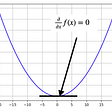# Gradient descent in Python from scratch

1. Some programming knowledge
2. A bit theoretical background — loss function, derivative, chain rule, etc.
3. Willingness to try out different stuffs
1. Open PyCharm
2. File -> New Project
3. Select Scientific from the left panel
4. Keep other settings as shown in the screenshot below, and click Create
`pip install numpy`
`import numpy as npimport mathimport matplotlib.pyplot as pltx = np.linspace(-math.pi, math.pi, 2000)y_sin = np.sin(x)plt.plot(x, y_sin)plt.show()`
`import numpy as npimport mathimport matplotlib.pyplot as pltx = np.linspace(-math.pi, math.pi, 2000)y_sin = np.sin(x)a = -0.025563539961665833b = 0.8777791634336599c = 0.004410136022528438d = -0.09632290690848559y_polynomial_cheat = a + b * x + c * x ** 2 + d * x ** 3plt.plot(x, y_sin)plt.plot(x, y_polynomial_cheat)plt.show()`
1. So, we are subtracting descent_grad_a from a. Let us try to understand how we are deriving descent_grad_a. Same, intuition can be applied for the other 3 parameters.
2. As we see in line#26, descent_grad_a is a product of grad_a and learning_rate. Let us first find out what grad_a is.
3. grad_a is gradient or derivative of the loss with respect to a, which in other words, a’s impact on the loss. Why there is a relation between derivatives and impact on change? That is kind of the definition of derivative — isn’t it?
1. Try different values of learning_rate
2. Try different values of the iterations
3. Try different loss functions
4. In the loop, print a,b,c,d, loss, and plot the graphs every time — or maybe in a regular interval (say, once in 100 iterations)
5. If you get comfortable with graph, try plotting loss with change in a,b,c,d
6. Set breakpoints in PyCharm and inspect variables.
7. Take a bigger range for y_sin, maybe -4π to +4π, and see if the approximation still works. If not, why not? (food for thought)

--

--

--

## More from Arif

PhD Student

Love podcasts or audiobooks? Learn on the go with our new app.

## ARM64 System Memory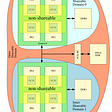## [Week 2, August 2021] Fleta Weekly Report## Extend those Native Classes!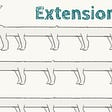## New Product! — How to send multiple transactions on the Binance network## A brief talk about technical debtPhD Student

## Intro to TensorFlow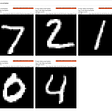## Linear Regression in Machine Learning: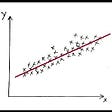## Let’s Learn: Neural Nets #3 — Activation Functions## Gradient Descent for “Everyone” | Accessible Machine Learning Series